# CBSE Previous Year Question Papers Class 12 Maths With Solutions

Here we are providing CBSE Previous Year Question Papers Class 6 to 12 solved with soutions CBSE Previous Year Question Papers Class 12 Maths with Solutions Maths sample paper class 12, Maths previous year question paper class 12, cbse class 12 Maths sample paper, cbse class 12 Maths sample paper 2020, Maths sample paper class 12 2020, cbse sample paper 2020 class 12 Maths, class 12 Maths sample paper 20 Practice of previous year question papers and sample papers protects each and every student to score bad marks in exams.If any student of CBSE Board continuously practices last year question paper student will easily score high marks in tests. Fortunately earlier year question papers can assist the understudies with scoring great in the tests. Unraveling previous year question paper class 12 Maths is significant for understudies who will show up for Class 12 Board tests.

## Class 12 Subject Maths Paper Set 3 with Solutions

SECTION A

Question numbers 1 to 20 carry 1 mark each.

Question numbers 1 to 10 are multiple choice type questions. Select the correct option.

Question 1: (Marks 1)

If f and g are two functions from R to R defined as f(x) = |x| + x and g(x) = |x| – x, then fog (x) for x < 0 is

(A) 4x

(B) 2x

(C) 0

(D) – 4x

Answer :

(D) –4x

Question 2: (Marks 1)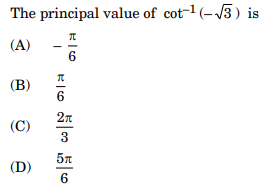Answer :Question 3: (Marks 1)Answer :

(A) 64

Question 4: (Marks 1)

The maximum value of slope of the curve y = – x 3 + 3x2 + 12x – 5 is

(A) 15

(B) 12

(C) 9

(D) 0

Answer :

(A) 15

Question 5: (Marks 1)Answer :

(A) tan (xex) + c

Question 6: (Marks 1)Answer :

(A) 1

Question 7: (Marks 1)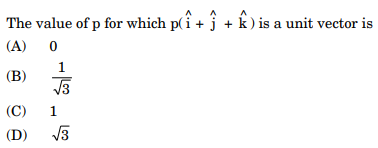Answer :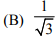Question 8: (Marks 1)

The coordinates of the foot of the perpendicular drawn from the point (– 2, 8, 7) on the XZ-plane is

(A) (– 2, – 8, 7)

(B) (2, 8, – 7)

(C) (– 2, 0, 7)

(D) (0, 8, 0)

Answer :

(C) (–2, 0, 7)

Question 9: (Marks 1)Answer :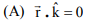Question 10: (Marks 1)Answer :

(B) (0, 8)

Fill in the blanks in question numbers 11 to 15

Question 11: (Marks 1)Answer :

0

ORQuestion 12: (Marks 1)Answer :Question 13: (Marks 1)

The equation of the tangent to the curve y = sec x at the point (0, 1) is __________ .

Answer :

y =1

Question 14: (Marks 1)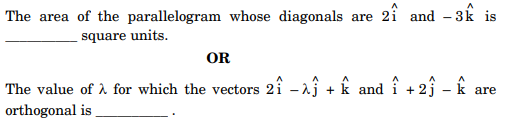Answer :

3

OR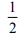Question 15: (Marks 1)

A bag contains 3 black, 4 red and 2 green balls. If three balls are drawn simultaneously at random, then the probability that the balls are of different colours is __________ .

Answer :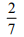Question numbers 16 to 20 are very short answer type questions.

Question 16: (Marks 1)

Construct a 2 × 2 matrix A = [aij] whose elements are given by aij = |(i)2 – j|.

Answer :Question 17: (Marks 1)

Differentiate sin2 (x) with respect to x.

Answer :Question 18: (Marks 1)

Find the interval in which the function f given by f(x) = 7 – 4x – x 2 is strictly increasing.

Answer :

f′ (x) 4 2x =− − 1/2

⇒ f(x) is increasing on (–∞, –2)

Question 19: (Marks 1)Answer :Question 20: (Marks 1)

An unbiased coin is tossed 4 times. Find the probability of getting at least one head.

Answer :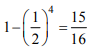SECTION B

Question numbers 21 to 26 carry 2 marks each.

Question 21: (Marks 2)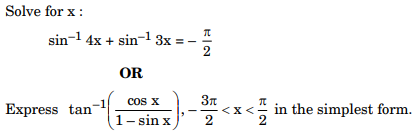Answer :Question 22: (Marks 2)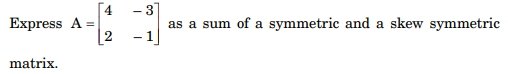Answer :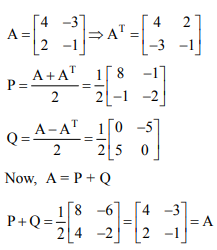Question 23: (Marks 2)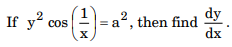Answer :Question 24: (Marks 2)Answer :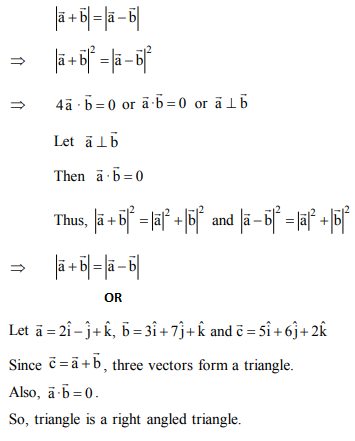Question 25: (Marks 2)

Find the coordinates of the point where the line through (– 1, 1, – 8) and (5, – 2, 10) crosses the ZX-plane.

Answer :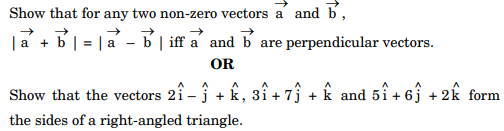Question 26: (Marks 2)

If A and B are two events such that P(A) = 0·4, P(B) = 0·3 and P(A ∪ B) = 0·6, then find P(B’ ∩ A)

Answer :

P(A ∩ B) = P(A) + P(B) − P(A ∪ B) 0.1 1

P(B’ ∩ A) = P(A) − P(A ∩ B) = 0.3

SECTION C

Question numbers 27 to 32 carry 4 marks each.

Question 27: (Marks 4)Answer :Question 28: (Marks 4)Answer :Question 29: (Marks 4)Answer :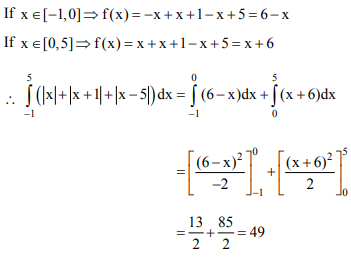Question 30: (Marks 4)

Find the general solution of the differential equation x 2y dx – (x3 + y3) dy = 0.

Answer :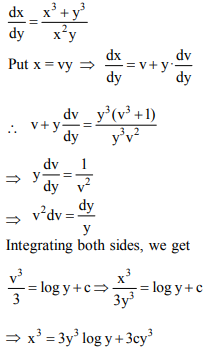Question 31: (Marks 4)

Solve the following LPP graphically :

Minimise z = 5x + 7y

subject to the constraints

2x + y ≥ 8

x + 2y ≥ 10

x, y ≥ 0

Answer :Question 32: (Marks 4)

A bag contains two coins, one biased and the other unbiased. When tossed, the biased coin has a 60% chance of showing heads. One of the coins is selected at random and on tossing it shows tails. What is the probability it was an unbiased coin ?

ORAnswer :SECTION D

Question numbers 33 to 36 carry 6 marks each.

Question 33: (Marks 4)

Solve the following system of equations by matrix method :

x – y + 2z = 7

2x – y + 3z = 12

3x + 2y – z = 5

OR

Obtain the inverse of the following matrix using elementary operations :Answer :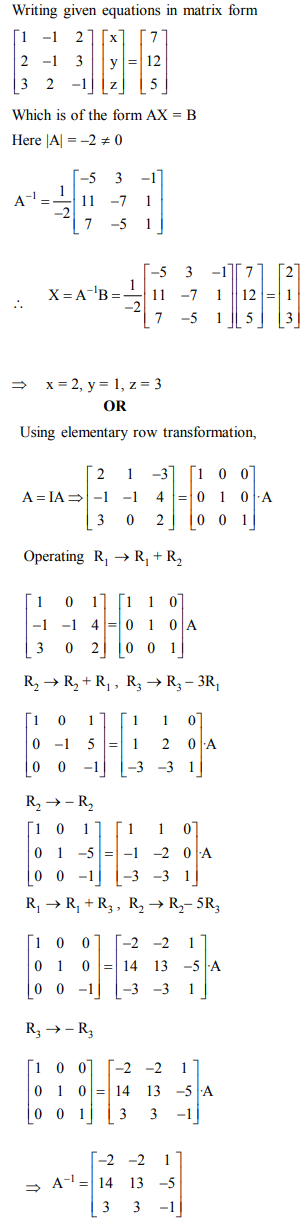Question 34: (Marks 4)

Find the points on the curve 9y2 = x2, where the normal to the curve makes equal intercepts with both the axes. Also find the equation of the normals.

Answer :Question 35: (Marks 4)

Find the area of the following region using integration: {(x, y) : y ≤ | x | + 2, y ≥ x2}.

OR

Using integration, find the area of a triangle whose vertices are (1,0), (2, 2) and (3,1).

Answer :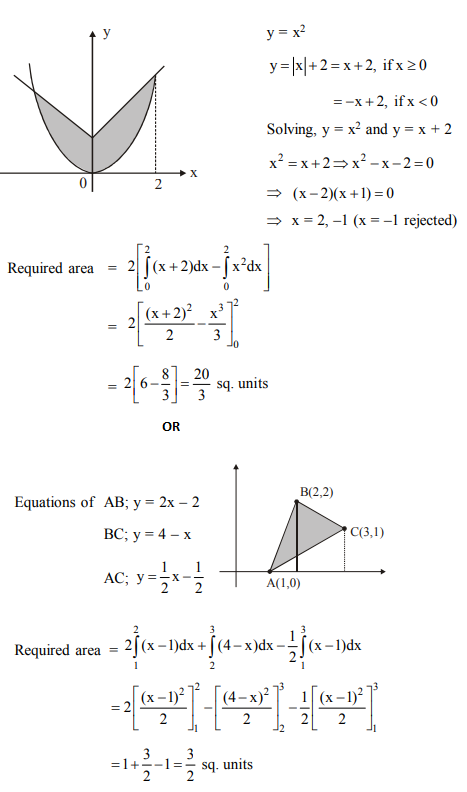Question 36: (Marks 4)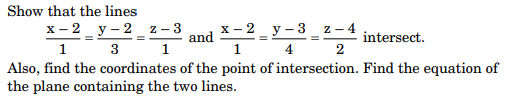Answer :☞ Click here for privious year Question papers

## Maths sample paper class 12, Maths previous year question paper class 12, cbse class 12 Maths sample paper, cbse class 12 Maths sample paper 2020, Maths sample paper class 12 2020, cbse sample paper 2020 class 12 Maths, class 12 Maths sample paper 2020, class 12 important questions Maths, cbse class 12 board exam Maths paper, Maths previous year question papers class 12 with solutions, Maths sample paper class 12 2019, cbse class 12 Maths question paper 2017 solved pdf, cbse class 12 Maths question paper 2018, class 12 Maths paper 2019, Maths question paper for class 12, cbse class 12 Maths paper 2019

### NCERT Books Free Pdf Download for Class 5, 6, 7, 8, 9, 10 , 11, 12 Hindi and English Medium

 Mathematics Biology Psychology Chemistry English Economics Sociology Hindi Business Studies Geography Science Political Science Statistics Physics Accountancy

## Please Share this webpage on facebook, whatsapp, linkdin and twitter.

Copyright @ ncerthelp.com A free educational website for CBSE, ICSE and UP board.